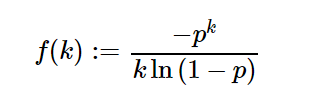Related Articles
sympy.stats.Logarithmic() in Python
• Last Updated : 08 Jun, 2020

With the help of `sympy.stats.Logarithmic()` method, we can get the random variable representing the logarithmic distribution.Syntax : `sympy.stats.Logarithmic(name, p)`
Return : Return the random variable.

Example #1 :
In this example we can see that by using `sympy.stats.Logarithmic()` method, we are able to get the random variable representing the logarithmic distribution by using this method.

 `# Import sympy and logarithmic ` `from` `sympy.stats ``import` `Logarithmic, density, E, variance ` `from` `sympy ``import` `Symbol, S ` ` `  `p ``=` `S.One ``/` `5` ` `  `# Using sympy.stats.Logarithmic() method ` `X ``=` `Logarithmic(``"x"``, p) ` `gfg ``=` `density(X)(``3``) ` ` `  `print``(gfg) `

Output :

-1/(375*log(4/5))

Example #2 :

 `# Import sympy and logarithmic ` `from` `sympy.stats ``import` `Logarithmic, density, E, variance ` `from` `sympy ``import` `Symbol, S ` ` `  `p ``=` `0.46` ` `  `# Using sympy.stats.Logarithmic() method ` `X ``=` `Logarithmic(``"x"``, p) ` `gfg ``=` `density(X)(``4``) ` ` `  `print``(gfg) `

Output :

0.0181660042052665

Attention geek! Strengthen your foundations with the Python Programming Foundation Course and learn the basics.

To begin with, your interview preparations Enhance your Data Structures concepts with the Python DS Course.

My Personal Notes arrow_drop_up
Recommended Articles
Page :